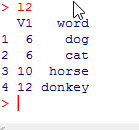# R: laply and ldply from plyr package

R is great programming language when it comes to manipulating data. That is one of the reasons it is so loved by data scientists and statisticians. Being an open source project, R also has the advantage of lots of additional packages that add even more functionality to the language.

The package I am focusing on today is the plyr package. I am just going to barely dip into this package, as I am only go to cover two functions from the package (laply and ldply).  I am covering these as I will be using them in a later lesson how to perform sentiment analysis on Twitter data.

```install.packages("plyr")
library(plyr)```

## The functions

laply takes in a list, applies a function, and exports the results into an array.

ldply takes in a list, applies a function, and exports the results into a dataframe.

The syntax for both is simple enough:

laply(list, function(x){ func.. })

We are going to do a simple example, creating a list of words and passing this list to a function that will count the characters in each string and then we will multiply the result times 2.

```#create data
l = list('dog','cat','horse','donkey')

l1 = laply(l, function(x){x1=nchar(x)
x1 = x1*2})```

If you now check l1, it will return an array of 6,6,10,12 (disclaimer – since this is a single column array  R actually places it into a simple vector)

Now let’s try ldply which return a dataframe ( more useful in my opinion)

```l2 = ldply(1, function(x) {x1 =nchar(x)*2})